Know more about the Repeaters Batch Know more about the Repeaters Batch

# JEE Advanced Question Paper 2021 Chemistry Paper 2

JEE Advanced 2021 Chemistry question paper for Paper 2 has been examined and solved by our subject experts. They have prepared the solutions accordingly and students can easily access them below. JEE Advanced 2021 Paper 2 Chemistry question paper solutions have been prepared in a step by step format and will enable students to develop the right approach to solve the different questions quickly.
The JEE Advanced 2021 Chemistry question paper uploaded at BYJU’S is a perfect study resource for candidates who are gearing up to appear for the entrance exam. By going through the question paper and solving the questions they will further get a clearer idea of the question paper pattern, question types and the difficulty level of the exam. It will also enable the students to obtain a fair idea about the distribution of marks with respect to each topic. All in all, practising these questions will help them boost their exam preparation and be ready to face one of the most competitive exams in India.

### JEE Advanced 2021 Paper 2 Chemistry Question Paper

Question 1. The reaction sequence(s) that would lead to o-xylene as the major product is(are).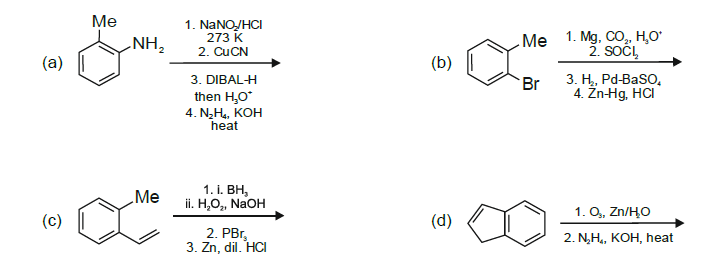Solution: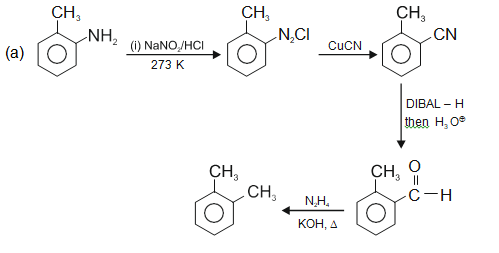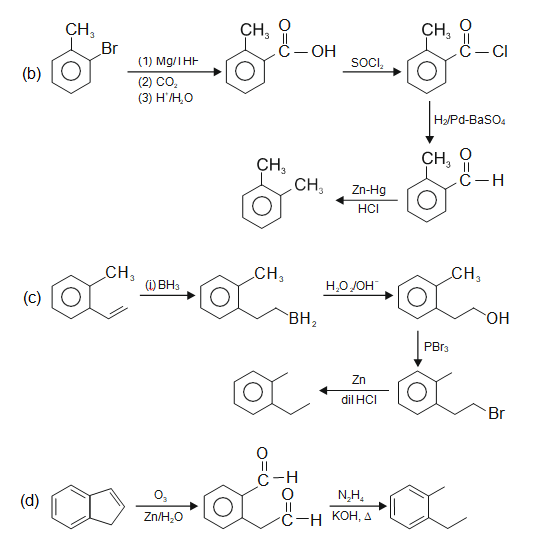Question 2. Correct option(s) for the following sequence of reactions is(are)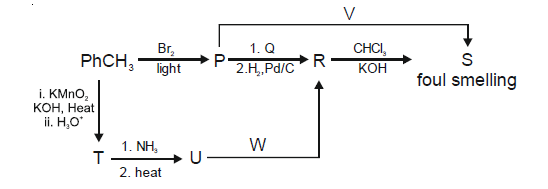1. a. Q = KNO2, W = LiAlH4
2. b. R = benzenamine, V = KCN
3. c. Q = AgNO2, R = phenylmethanamine
4. d. W = LiAlH4, V = AgCN

Solution: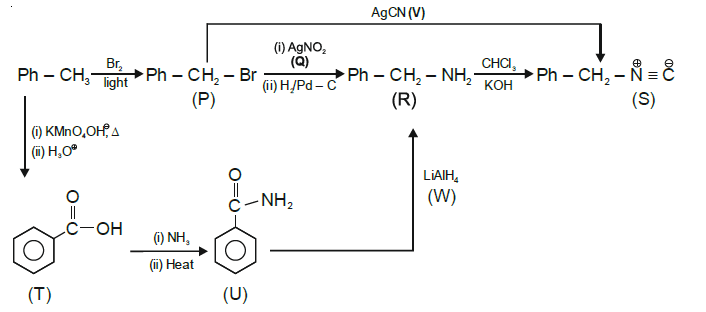Therefore, correct options are

Q = AgNO2, R = phenylmethanamine

W = LiAlH4, V = AgCN

Question 3. For the following reaction;

$$2X + Y\overset{k}{\rightarrow}P$$

The rate of reaction is

$$\frac{d[P]}{dt} = k[X]$$
. Two moles of X are mixed with one mole of Y to make 1.0 L of solution. At 50 s, 0.5 mole of Y is left in the reaction mixture. The correct statement(s) about the reaction is(are). (Use: ln 2 = 0.693)

a. The rate constant, k, of the reaction is 13.86 × 10-4 s-1.

1. a. The rate constant, k, of the reaction is 13.86 × 10-4 s-1.
2. b. Half-life of X is 50 s.
3. c. At 50 s, - d[X] / dt = 13.86 × 10-3 mol L-1 s-1.
4. d. At 100 s, d[Y] / dt = 3.46 × 10-3 mol L-1 s-1.

Solution:

rate =

$$\frac{d[P]}{dt} = k[X]$$

2X + Y → P

2 mole 1 mole

1 mole 0.5 mole 0.5 mole

- d[X] / dt = k1[X] = 2k[X] ⇒ 2k = k1

- d[Y] / dt = k2[X] = 2k[X] ⇒ k2 = k

2K = 1/50 In2

K = 1 / 100 In2 = 0.6 93 / 100 = 6.93 × 10-3 × s-1 = 50 sec

At 50 sec,

d[X] / dt = 2k[X] = 2 × 0.693 / 100 × 1

= 13.86 × 10-3 mol L-1 s-1

At 100 sec

-d[Y] / dt = k2[X] = k[X] × 0.693 / 100 × ½

(Concentration of X after 2 half-lives = ½ M)

= 3.46 × 10-3 mol L-1 s-1

Question 4. Some standard electrode potentials at 298 K are given below:

Pb2+/Pb –0.13 V

Ni2+/Ni –0.24 V

C2+/Cd –0.40 V

Fe2+/Fe –0.44 V

To a solution containing 0.001 M of X2+ and 0.1 M of Y2+, the metal rods X and Y are inserted (at 298 K) and connected by a conducting wire. This resulted in the dissolution of X.

The correct combination(s) of X and Y, respectively, is(are)

(Given: Gas constant, R = 8.314 J K-1 mol-1, Faraday constant, F = 96500 C mol-1)

1. a. Cd and Ni
2. b. Cd and Fe
3. c. Ni and Pb
4. d. Ni and Fe

Solution:

X + Y2+ X2+ + Y

$$E = E^{0} - \frac{0.06}{2} log_{10}\left ( \frac{10^{-3}}{10^{-1}} \right )$$

E = Eo + 0.06

(a) E° = –(– .4) + (– .24) = .16 > 0

(b) E° = –(– .4) + (– .44) = –.04 < 0 and Ecell = –0.04 + 0.06 = +0.02 > 0

(c) E° = –(– .24) + (– .13) = .11 > 0

(d) E° = –(– .24) + (– .44) = –.2 < 0

∴ Ecell = –0.2 + 0.06 = –0.14 < 0

∴ If Ecell > 0 then the cell construction is possible.

Question 5. The pair(s) of complexes wherein both exhibit tetrahedral geometry is(are) (Note: py = pyridine, Given: Atomic numbers of Fe, Co, Ni and Cu are 26, 27, 28 and 29, respectively)

1. a. [FeCl4] and [Fe(CO)4]2–
2. b. [Co(CO)4] and [CoCl4]2–
3. c. [Ni(CO)4] and [Ni(CN)4]2–
4. d. [Cu(py)4]+ and [Cu(CN)4]3–

Solution:

[FeCl4] → Fe3+, 3d5 (weak field ligand) = sp3

[Fe(CO)4]–2 → Fe2–, 3d10 →sp3

[Co(CO)4] → Co, 3d10 → sp3

[CoCl4]2– → Co2+, 3d7 (weak field ligand) → sp3

[Ni(CO)4] → Ni, 3d10 → sp3

[Ni(CN)4]2– → Ni2+, 3d8 (strong field ligand) → dsp2

[Cu(py)4]+ → Cu+, 3d10 →sp3

[Cu(CN)4]3– → Cu+, 3d10 → sp3

In 3d10 electronic configuration, only sp3 hybridisation and tetrahedral geometry are possible.

Question 6. The correct statement(s) related to oxoacids of phosphorous is(are).

1. a. Upon heating, H3PO3 undergoes a disproportionation reaction to produce H3PO4 and PH3.
2. b. While H3PO3 can act as a reducing agent, H3PO4 cannot.
3. c. H3PO3 is a monobasic acid.
4. d. The H atom of the P-H bond in H3PO3 is not ionizable in water.

Solution:

$$4H_{3}PO_{3} \overset{\Delta }{\rightarrow}PH_{3} + 3H_{3}PO_{4}$$

In H3PO4, phosphorous is present in the highest oxidation state, i.e., +5. So H3PO4 cannot act as a reducing agent. Structure of H3PO3,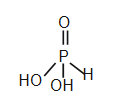It is a dibasic acid.

H atom present in the P–H bond is not ionizable.

These P-H bonds are not ionisable to give H+ and do not play any role in basicity. Only those H atoms which are attached with oxygen in P-OH form are ionisable and cause the basicity. Thus, H3PO3 and H3PO4 are dibasic and tribasic, respectively as the structure of H3PO3 has two P – OH bonds and H3PO4 three.

Question Statement for Questions 7 and 8.

At 298 K, the limiting molar conductivity of a weak monobasic acid is 4 × 102 S cm2 mol–1. At 298 K, for an aqueous solution of the acid, the degree of dissociation is a and the molar conductivity is y × 102 S cm2 mol–1. At 298 K, upon 20 times dilution with water, the molar conductivity of the solution becomes 3y × 102 S cm2 mol–1.

Question 7. The value of α is _______.

Solution:

Question 8. The value of y is _______.

Solution:

Solution for Questions 7 and 8.

Molar conductivity of HX at infinite dilution

$$\Lambda _{m}^{\infty }$$
= 4 × 102 S cm2 mol-1

Molar conductivity of HX at conc. c1 = y × 102 S cm2 mol-1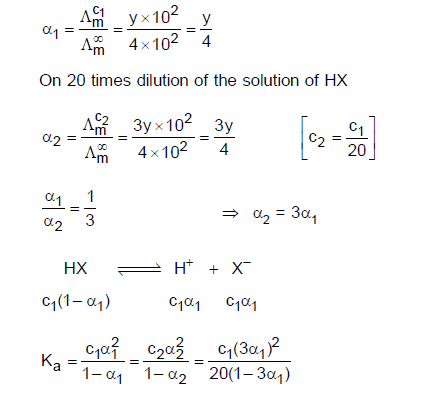$$\frac{1}{1-\alpha _{1}} = \frac{9}{20(1-3\alpha _{1})}$$

20 - 60α1 = 9 - 9α1

⇒ α1 = 11/51 = 0.215

Y = 4α1 = 0.086

Question Statement for Questions 9 and 10.

The reaction of x g of Sn with HCl quantitatively produced a salt. The entire amount of the salt reacted with y g of nitrobenzene in the presence of the required amount of HCl to produce 1.29 g of an organic salt (quantitatively).

(Use Molar masses (in g mol–1) of H, C, N, O, Cl and Sn as 1, 12, 14, 16, 35 and 119, respectively).

Question 9. The value of x is ________.

Solution:

Sn + HCl → SnCl2

⇒ Moles of ammonium salt = 1.29 / 129 = 0.01

⇒ Moles of nitrobenzene = 0.01

No. of eq. of nitrobenzene = No. of eq. of SnCl2

6 × (0.01) = 2 ×

$$n_{SnCl_{2}}$$

$$n_{SnCl_{2}}$$
= 0.03

⇒ nSn = 0.03

wSn = 0.03 × 119

x = 3.57

Question 10. The value of y is ________.

Solution:

Solution of Question Nos. 9 and 10

Sn + HCl → SnCl2

⇒ Moles of ammonium salt = 1.29 / 129 = 0.01

⇒ Moles of nitrobenzene = 0.01

⇒ y = 0.01 × Molar mass of nitrobenzene

= 0.01 × 123

= y = 1.23

Question Statement for Questions 11 and 12.

A sample (5.6 g) containing iron is completely dissolved in cold dilute HCl to prepare a 250 mL of solution. Titration of 25.0 mL of this solution requires 12.5 mL of 0.03 M KMnO4 solution to reach the endpoint. Number of moles of Fe2+ present in 250 mL solution is x × 10–2 (consider complete dissolution of FeCl2). The amount of iron present in the sample is y% by weight.

(Assume: KMnO4 reacts only with Fe2+ in the solution Use: Molar mass of iron as 56 g mol–1)

Question 11. The value of x is ________.

1. a.swer: (1.875)

Solution:

Question 12. The value of y is ________.

Solution:

Solution of Question Nos. 11 and 12

8H+ + 5Fe2+ + MnO → 5Fe3+ + Mn2+ + 4H2O

For 25 ml,

meq of Fe2+ = meq of MnO

= 12.5 × 0.03 × 5

For 250 ml,

mmoles of Fe2+ = 12.5 × 0.03 × 5 × 250 / 25

moles of Fe2+ = 18.75 / 1000 mol

= 18.75 × 10–3 mol

= 1.875 × 10–2 mol

x = 1.875

Weight of Fe2+ = 1.875 × 10–2 × 56 = 1.05 g

% purity of Fe2+ y = 18.75%

= 1.05 / 5.6 ×100

Statement: The amount of energy required to break a bond is the same as the amount of energy released when the same bond is formed. In a gaseous state, the energy required for homolytic cleavage of a bond is called Bond Dissociation Energy (BDE) or Bond Strength. BDE is affected by the s-character of the bond and the stability of the radicals formed. Shorter bonds are typically stronger bonds. BDEs for some bonds are given below: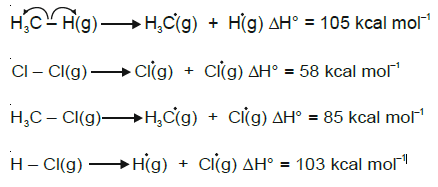Question 13. The correct match of the C-H bonds (shown in bold) in Column J with their BDE in Column K is;

Column J Column K
Molecule BDE (kcal mol–1)
(P) H-CH(CH3)2 (i) 132
(Q) H-CH2Ph (ii) 110
(R) H-CH=CH2 (iii) 95
(S) H-CºCH (iv) 88

1. a. P – iii, Q – iv, R – ii, S – i
2. b. P – i, Q – ii, R – iii, S – iv
3. c. P – iii, Q – ii, R – i, S – iv
4. d. P – ii, Q – i, R – iv, S – iii

Solution: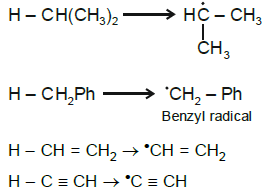Order of stability of free radical

Q > P > R > S

Stability of free radical α 1 / Bond energy

∴ Order of bond energy :

S > R > P > Q

Question 14. For the following reaction,

$$CH_{4} (g) + Cl_{2} (g) \overset{light}{\rightarrow}CH_{3}Cl(g) + HCl(g)$$

the correct statement is;

1. a. Initiation step is exothermic with DH° = –58 kcal mol–1
2. b. Propagation step involving ·CH3 formation is exothermic with DH° = –2 kcal mol–1
3. c. Propagation step involving CH3Cl formation is endothermic with DH° = +27 kcal mol–1
4. d. The reaction is exothermic with DH° = –25 kcal mol–1

Solution: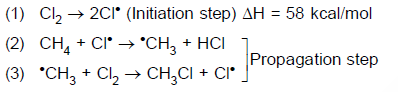Step (1) → Endothermic (bond breaking)

Step (2) → ∆H = 105 – 103 = 2 kcal/mol (Endothermic)

Step (3) → ∆H = 58 – 85 = –27 kcal/mol (Exothermic)

For complete reaction

$$CH_{4} (g) + Cl_{2} (g) \overset{light}{\rightarrow}CH_{3}Cl(g) + HCl(g)$$

∆H = 58 + 105 – 85 – 103

= –25 kcal/mol

Question Statement for Questions 15 and 16.

The reaction of K3[Fe(CN)6] with freshly prepared FeSO4 solution produces a dark blue precipitate called Turnbull's blue. The reaction of K4[Fe(CN)6] with the FeSO4 solution in the complete absence of air produces a white precipitate X, which turns blue in the air. Mixing the FeSO4 solution with NaNO3, followed by slow addition of concentrated H2SO4 through the side of the test tube produces a brown ring.

Question 15. Precipitate X is

1. a. Fe4[Fe(CN)6]3
2. b. Fe4[Fe(CN)6]
3. c. K2Fe[Fe(CN)6]
4. d. KFe[Fe(CN)6]

Solution:

Question 16. Among the following, the brown ring is due to the formation of

1. a. [Fe(NO)2(SO4)2]2–
2. b. [Fe(NO)2(H2O)4]3+
3. c. [Fe(NO)4(SO4)2]
4. d. [Fe(NO)(H2O)5]2

Solution:

Solution of Question Nos. 15 and 16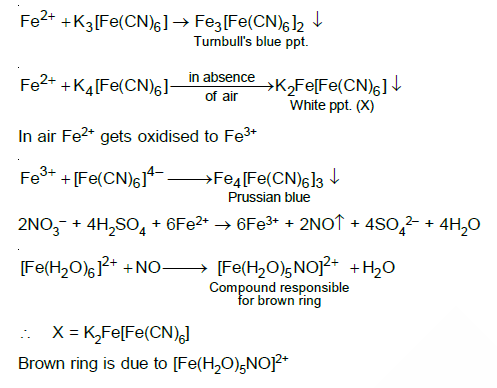Question 17. One mole of an ideal gas at 900 K, undergoes two reversible processes, I followed by II, as shown below. If the work done by the gas in the two processes are the same, the value of

$$In\frac{v_{3}}{v_{2}}$$
is ____.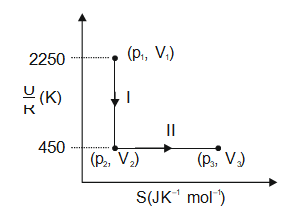(U: internal energy, S: entropy, p: pressure, V: volume, R: gas constant)

(Given: molar heat capacity at constant volume, C of the gas is 5 / 2 R)

Solution:

Process II is a reversible isothermal process

∆U / R = 450 - 2250

∆U = -1800 R

WI = ∆U = -1800R

Process II - (Reversible Isothermal Process)

T1 = 900 K

Calculation of T2 after the reversible adiabatic process

–1800R = nCv(T2 – T1)

–1800R 1 × 5/2 R(T2 – 900)

T2 = 180 K

WII = –nRT2 In = W

–1 × R × 180ln v3 / v2 –1800R

ln v3 / v210

Question 18. Consider a helium (He) atom that absorbs a photon of wavelength 330 nm. The change in the velocity (in cm s-1) of the He atom after the photon absorption is_____.

(Assume: Momentum is conserved when the photon is absorbed.

Use: Planck constant = 6.6 × 10-34 J s, Avogadro number = 6 × 1023 mol-1, Molar mass of He = 4 g mol-1)

Solution:

Momentum of photon =

$$\frac{h}{\lambda} = \frac{6.6\times 10^{-27}}{330\times 10^{-7}}$$
gm cm s-1

Momentum of 1 mole of He-atoms = m∆v

∴ m∆v = NA × h / λ

$$4\times \Delta v = \frac{6\times10^{23} \times 6.6\times 10^{-27}}{330\times 10^{-7}}$$

$$\Delta v = \frac{6\times 6.6\times 10^{2}}{33\times 4}$$
= 30 cm s-1

∴ Change in velocity of He-atoms = 30 cm s-1

Question 19. Ozonolysis of ClO2 produces oxide of chlorine. The average oxidation state of chlorine in this oxide is ____.

Solution:

ClO2 contains an odd electron and is paramagnetic. It reacts with ozone to give O2 and Cl2O6.

2ClO2 + 2O3 → Cl2O6 + 2O2

In Cl2O6, the average oxidation state of Cl is +6.

### JEE Advanced 2021 Chemistry Paper 2 Solutions